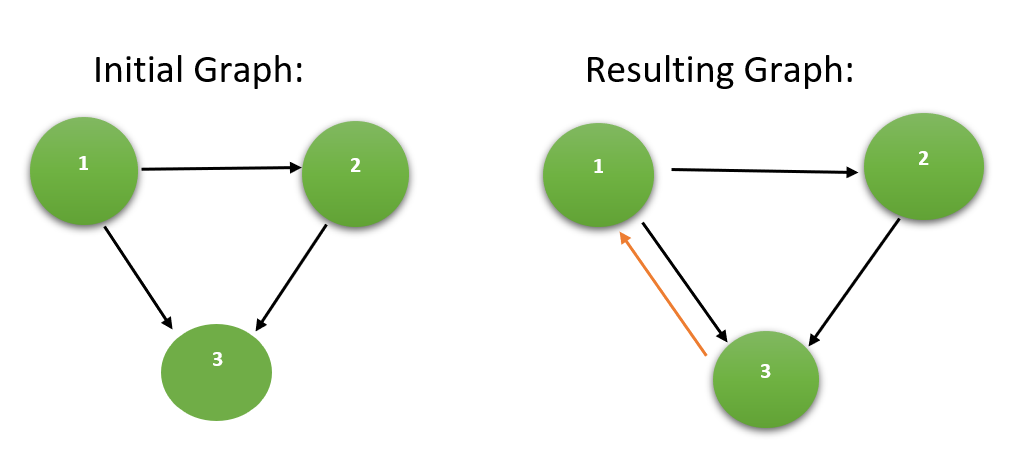Minimum edges required to make a Directed Graph Strongly Connected

• Difficulty Level : Hard
• Last Updated : 23 Oct, 2021

Given a Directed graph of N vertices and M edges, the task is to find the minimum number of edges required to make the given graph Strongly Connected.

Examples:

Input: N = 3, M = 3, source[] = {1, 2, 1}, destination[] = {2, 3, 3}
Output:
Explanation:
Adding a directed edge joining the pair of vertices {3, 1} makes the graph strongly connected.
Hence, the minimum number of edges required is 1.
Below is the illustration of the above example:Input: N = 5, M = 5, source[] = {1, 3, 1, 3, 4}, destination[] = {2, 2, 3, 4, 5}
Output:
Explanation:
Adding 2 directed edges to join the following pair of vertices makes the graph strongly connected:

• {2, 1}
• {5, 2}

Hence, the minimum number of edges required is 2.

Approach:
For a Strongly Connected Graph, each vertex must have an in-degree and an out-degree of at least 1. Therefore, in order to make a graph strongly connected, each vertex must have an incoming edge and an outgoing edge. The maximum number of incoming edges and the outgoing edges required to make the graph strongly connected is the minimum edges required to make it strongly connected.
Follow the steps below to solve the problem:

• Find the count of in-degrees and out-degrees of each vertex of the graph, using DFS.
• If the in-degree or out-degree of a vertex is greater than 1, then consider it as only 1.
• Count the total in-degree and out-degree of the given graph.
• The minimum number of edges required to make the graph strongly connected is then given by max(N-totalIndegree, N-totalOutdegree).
• Print the count of minimum edges as the result.

Below is the implementation of the above approach:

C++

 // C++ program to implement// the above approach#include using namespace std;  // Perform DFS to count the in-degree// and out-degree of the graphvoid dfs(int u, vector adj[], int* vis, int* inDeg,         int* outDeg){    // Mark the source as visited    vis[u] = 1;        // Traversing adjacent nodes    for (auto v : adj[u])    {        // Mark out-degree as 1        outDeg[u] = 1;        // Mark in-degree as 1        inDeg[v] = 1;          // If not visited        if (vis[v] == 0)        {            // DFS Traversal on            // adjacent vertex            dfs(v, adj, vis, inDeg, outDeg);        }    }}  // Function to return minimum number// of edges required to make the graph// strongly connectedint findMinimumEdges(int source[], int N, int M, int dest[]){    // For Adjacency List    vector adj[N + 1];      // Create the Adjacency List    for (int i = 0; i < M; i++)    {        adj[ source[i] ].push_back(dest[i]);    }      // Initialize the in-degree array    int inDeg[N + 1] = { 0 };      // Initialize the out-degree array    int outDeg[N + 1] = { 0 };      // Initialize the visited array    int vis[N + 1] = { 0 };      // Perform DFS to count in-degrees    // and out-degreess    dfs(1, adj, vis, inDeg, outDeg);      // To store the result    int minEdges = 0;      // To store total count of in-degree    // and out-degree    int totalIndegree = 0;    int totalOutdegree = 0;      // Find total in-degree    // and out-degree    for (int i = 1; i <= N; i++)    {        if (inDeg[i] == 1)            totalIndegree++;        if (outDeg[i] == 1)            totalOutdegree++;    }      // Calculate the minimum    // edges required    minEdges = max(N - totalIndegree, N - totalOutdegree);      // Return the minimum edges    return minEdges;}  // Driver Codeint main(){    int N = 5, M = 5;      int source[] = { 1, 3, 1, 3, 4 };    int destination[] = { 2, 2, 3, 4, 5 };      // Function call    cout << findMinimumEdges(source, N, M, destination);    return 0;}

Python3

 # Python3 program to implement# the above approach   # Perform DFS to count the in-degree# and out-degree of the graphdef dfs(u, adj, vis,inDeg, outDeg):      # Mark the source as visited    vis[u] = 1;       # Traversing adjacent nodes    for v in adj[u]:        # Mark out-degree as 1        outDeg[u] = 1;        # Mark in-degree as 1        inDeg[u] = 1;                  # If not visited        if (vis[v] == 0):               # DFS Traversal on            # adjacent vertex            dfs(v, adj, vis,                 inDeg, outDeg)  # Function to return minimum # number of edges required # to make the graph strongly # connecteddef findMinimumEdges(source, N,                      M, dest):      # For Adjacency List    adj = [[] for i in range(N + 1)]       # Create the Adjacency List    for i in range(M):        adj].append(dest[i]);             # Initialize the in-degree array    inDeg = [0 for i in range(N + 1)]       # Initialize the out-degree array    outDeg = [0 for i in range(N + 1)]       # Initialize the visited array    vis = [0 for i in range(N + 1)]       # Perform DFS to count in-degrees    # and out-degreess    dfs(1, adj, vis, inDeg, outDeg);       # To store the result    minEdges = 0;       # To store total count of     # in-degree and out-degree    totalIndegree = 0;    totalOutdegree = 0;       # Find total in-degree    # and out-degree    for i in range(1, N):            if (inDeg[i] == 1):            totalIndegree += 1;        if (outDeg[i] == 1):            totalOutdegree += 1;           # Calculate the minimum    # edges required    minEdges = max(N - totalIndegree,                    N - totalOutdegree);       # Return the minimum edges    return minEdges;  # Driver codeif __name__ == "__main__":          N = 5    M = 5       source = [1, 3, 1, 3, 4]    destination = [2, 2, 3, 4, 5]       # Function call    print(findMinimumEdges(source, N,                            M, destination))      # This code is contributed by rutvik_56

Javascript


Output
2

Time Complexity: O(N + M)
Auxiliary Space: O(N)

My Personal Notes arrow_drop_up